## 13.5.1 Differential Decision Making

To make a differential game against nature that extends Formulation 10.1 to continuous time, suppose that nature actions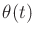are chosen from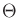. A differential model can be defined as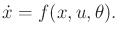(13.199)

The state spaceand action space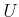are used in the same way as throughout this chapter. The difference only comes in the state transition equation. State-dependent nature action spaces may also be used.

As observed repeatedly throughout Part III, nature can be modeled nondeterministically or probabilistically. In the nondeterministic case, (13.199) is equivalent to a differential inclusion :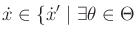such that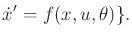(13.200)

Possible future values for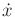can be computed using forward projections. Reachable sets, which will be introduced in Section 14.2.1, can be defined that characterize the evolution of future possible states over time. Plans constructed under this model usually use worst-case analysis.

Example 13..15 (Nondeterministic Forward Projection)   As a simple example of using (13.199), consider expressing the uncertainty model used in the preimage planning framework of Section 12.5.1.

At each time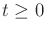, nature chooses some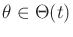. The state transition equation is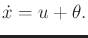(13.201)

The cone shown in Figure 12.45 is just the nondeterministic forward projection under the application of a constant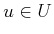.In the probabilistic case, restrictions must be carefully placed on the nature action trajectory (e.g., a Weiner process ). Under such conditions, (13.199) becomes a stochastic differential equation. Planning in this case becomes continuous-time stochastic control , and the task is to optimize the expected cost.

Example 13..16 (A Simple Car and Nature)   Uncertainty can be introduced into any of the models of this chapter. For example, recall the simple car, (13.15). Suppose that nature interferes with the steering action so that it is not precisely known in which direction the car will drive. Let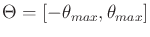, in which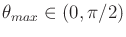represents the maximum amount of steering angle error that can be caused by nature. The simple-car model can be modified to account for this error as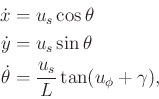(13.202)

in which the domain of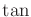must be extended toor other suitable restrictions must be imposed. At each time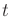, a nature action13.12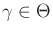causes the true heading of the car to be perturbed from the commanded direction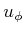. Under nondeterministic uncertainty, the maximum amount that the car deviates from the commanded direction must be determined by the planning algorithm. A probability density function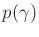can be assigned to obtain a probabilistic model. When integrated over time, (13.202) yields probability density functions over future car configurations .In a similar way, parameters that account for nature can be introduced virtually anywhere in the models of this chapter. Some errors may be systematic, which reflect mistakes or simplifications made in the modeling process. These correspond to a constant nature action applied at the outset. In this case, nature is not allowed to vary its action over time. Other errors could correspond to noise, which is expected to yield different nature actions over time.

Steven M LaValle 2020-08-14